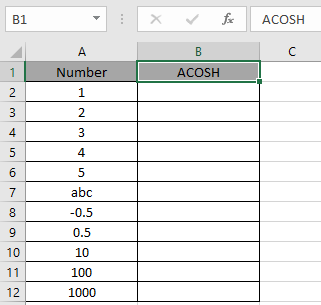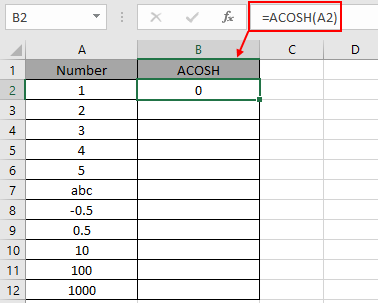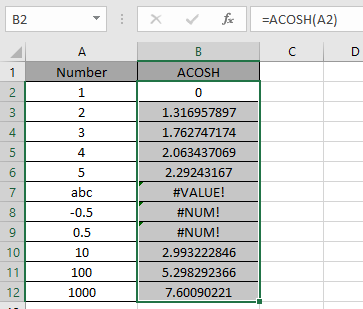# How to use the ACOSH Function in Excel

COSH function is a Mathematical function to calculate the hyperbolic Cosine of the value. COSH function is built in excel function. Hyperbolic function of COSH defined using the exponential function.

Cosh (x) = (ex+ e-x) / 2

Where ex = exponential function , e = 2.71828

So, it means Cosine hyperbolic function returns the value as the formula stated above. Now if we wish to return the inverse of Cosine hyperbolic function, excel ACOSH function does our work. The below stated

x =  COSH -1(ex+ e-x) / 2    { in mathematics }

x =  ACOSH [(ex+ e-x) / 2]   { in Excel }

So, The inverse hyperbolic Cosine is the value whose hyperbolic Cosine is the number, so ACOSH(COSH(number)) equals number.

ACOSH ( COSH ( number ) ) = number

ACOSH function in excel returns inverse of the Cosine hyperbolic function in excel. The

Syntax:

= ACOSH ( number )

number : input any real number equal to greater than 1

Let’s understand this function using it in an example.Here we have values which will be input to the function and we need to find the inverse of the hyperbolic Cosine value using the ACOSH function in excel
Use the formula:

=COSH ( A2 )

A2 : number given as cell reference.Here the argument to the function is provided as cell reference. As shown in the above snapshot, the Resultant comes out to be 0.

The value will calculated  using the mathematical formulation stated :

x = COSH -1 (e1+ e-1) / 2  = COSH -1[( e + 1/e ) / 2] = 0

Now copy the formula to other cells using the Ctrl + D shortcut key.Excel calculates the inverse of the Hyperbolic Cosine of a number using the COSH function.

Here are some of the observations gathered using the ACOSH function

Note:

1. The function returns #VALUE! Error if the argument to the function is non - numeric.
2. The function returns #NUM error, if the argument number is less than 1.
3. The input argument to the function can be any real number.
4. Argument to the function can be provided directly or using the cell reference in excel.

As you can see, we can get the inverse of the Cosine hyperbolic function using ACOSH function.

Hope you understood How to use ACOSH function in Excel. Explore more articles on Mathematical function like ASINH function and ATANH function here. Please state your query in the comment box below.

Related Articles

How to use the COSH function in Excel

How to use the COS function in Excel

How to use Wildcards in Excel

Popular Articles

Edit a dropdown list

If with conditional formatting

If with wildcards

Vlookup by date

Terms and Conditions of use

The applications/code on this site are distributed as is and without warranties or liability. In no event shall the owner of the copyrights, or the authors of the applications/code be liable for any loss of profit, any problems or any damage resulting from the use or evaluation of the applications/code.